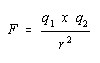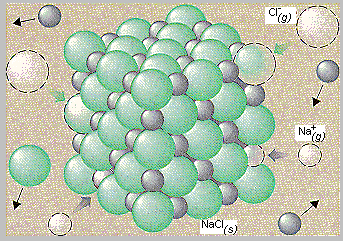Lattice Energy

Lattice Energies and the Strength of the Ionic Bond

The force of attraction between oppositely charged particles is directly proportional to the product of the charges on the two objects (q1 and q2) and inversely proportional to the square of the distance between the objects (r2).The strength of the bond between the ions of opposite charge in an ionic compound therefore depends on the charges on the ions and the distance between the centers of the ions when they pack to form a crystal.

An estimate of the strength of the bonds in an ionic compound can be obtained by measuring the lattice energy of the compound, which is the energy given off when oppositely charged ions in the gas phase come together to form a solid.

Example: The lattice energy of NaCl is the energy given off when Na+ and Cl- ions in the gas phase come together to form the lattice of alternating Na+ and Cl- ions in the NaCl crystal shown in the figure below.

 Na+(g) + Cl-(g)NaCl(s)Ho = -787.3 kJ/molThe lattice energies of ionic compounds are relatively large. The lattice energy of NaCl, for example, is 787.3 kJ/mol, which is only slightly less than the energy given off when natural gas burns.

The bond between ions of opposite charge is strongest when the ions are small.

The lattice energies for the alkali metal halides is therefore largest for LiF and smallest for CsI, as shown in the table below.

Lattice Energies of Alkali Metals Halides (kJ/mol)

 F- Cl- Br- I- Li+ 1036 853 807 757 Na+ 923 787 747 704 K+ 821 715 682 649 Rb+ 785 689 660 630 Cs+ 740 659 631 604

The ionic bond should also become stronger as the charge on the ions becomes larger. The data in the table below show that the lattice energies for salts of the OH- and O2- ions increase rapidly as the charge on the ion becomes larger.

Lattice Energies of Salts of the OH- and O2- Ions (kJ/mol)

 OH- O2- Na+ 900 2481 Mg2+ 3006 3791 Al3+ 5627 15,916Lattice Energies and Solubility

When a salt, such as NaCl dissolves in water, the crystals disappear on the macroscopic scale. On the atomic scale, the Na+ and Cl- ions in the crystal are released into solution.

 NaCl(s) H2ONa+(aq) + Cl-(aq)

The lattice energy of a salt therefore gives a rough indication of the solubility of the salt in water because it reflects the energy needed to separate the positive and negative ions in a salt.

Sodium and potassium salts are soluble in water because they have relatively small lattice energies. Magnesium and aluminum salts are often much less soluble because it takes more energy to separate the positive and negative ions in these salts. NaOH, for example, is very soluble in water (420 g/L), but Mg(OH)2 dissolves in water only to the extent of 0.009 g/L, and Al(OH)3 is essentially insoluble in water.